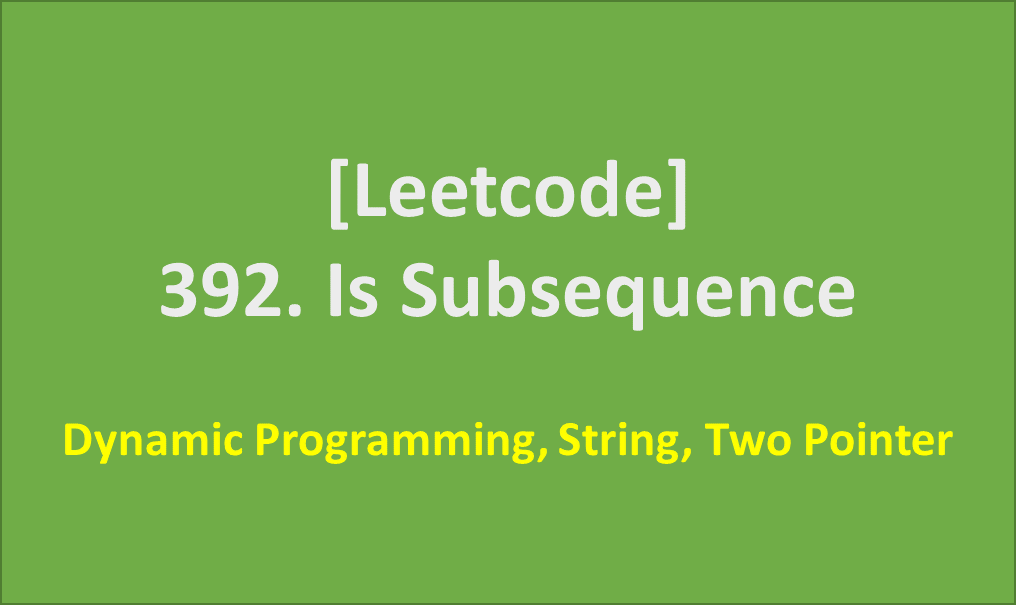# [Leetcode]392. Is Subsequence

limelimejiwon·2022년 4월 8일
0

## Python으로 공부하는 코딩테스트

목록 보기
36/67## 📄 Description

Given two strings s and t, return true if s is a subsequence of t, or false otherwise.

A subsequence of a string is a new string that is formed from the original string by deleting some (can be none) of the characters without disturbing the relative positions of the remaining characters. (i.e., "ace" is a subsequence of "abcde" while "aec" is not).

### Example 1:

Input: s = "abc", t = "ahbgdc"
Output: true

### Example 2:

Input: s = "axc", t = "ahbgdc"
Output: false

### Constraints:

• 0 <= s.length <= 100
• 0 <= t.length <= 104
• s and t consist only of lowercase English letters.

## 🔨 My Solution

• using Two Pointers

## 💻 My Submission

class Solution:
def isSubsequence(self, s: str, t: str) -> bool:
p1=p2=0
if len(s)==0: return True

while p1<len(s) and p2<len(t):
if s[p1]==t[p2]:
p1+=1
p2+=1

if p1==len(s): return True
return False

## Complexity

Time Complexity: O(n)
Space Complexity: O(1)

Suppose there are lots of incoming s, say s1, s2, ..., sk where k >= $10^9$, and you want to check one by one to see if t has its subsequence. In this scenario, how would you change your code?

## ❗ Follow up - 첫 번째 시도: 실패

class Solution:
def isSubsequence(self, s: str, t: str) -> bool:
# 순서 고려 안됨...!!
s_count=Counter(s)
t_count=Counter([x for x in t if x in s])

if s_count==t_count: return True
return False

### why?

• 개수만 비교할 뿐, 순서가 고려되지 않았다.

References